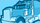cancel
Showing results for
Did you mean:

## integration to authorize.net

Hi ,

I am trying to integrate Authorize.net with  saleforce.com using SIM mathod but i am not able to create macthing fingarprint for authorize.net .i am getting error (99) This transaction cannot be accepted.

The server-generated fingerprint does not match the merchant-specified fingerprint in the x_fp_hash field.

i am not able to generate  fingerprint using force.com APEX. can  any one suggest me  what to do in this condition?Member
1 ACCEPTED SOLUTION

Accepted Solutions

thanks i solved it.  my code is ok  i was mistaken on last line of the code

String macUrl =EncodingUtil.urlEncode(EncodingUtil.base64Encode(mac), 'UTF-8');

it is

String macUrl =EncodingUtil.convertToHex(mac);

now it is working fine ..6 REPLIES 6

All you have to do is write a function that duplicates the following (modified from the PHP API to be a bit more readable):

```public static function getFingerprint(\$api_login_id, \$transaction_key, \$amount, \$fp_sequence, \$fp_timestamp) {

\$transaction_key =
(\$transaction_key ? \$transaction_key :
(defined('AUTHORIZENET_TRANSACTION_KEY') ? AUTHORIZENET_TRANSACTION_KEY : ''));

return (function_exists('hash_hmac') ?
hash_hmac('md5', \$raw) :
bin2hex(mhash(MHASH_MD5, \$raw)));
}```

The  key part is the last few lines.Expert

can you tell me how can i encrypt this in Force.com Apex. When i tryied in Apex it is giving me error Fingur prints dont match?Paste the code you're using to try to create the fingerprint, preferably in a code box (fourth button from left in Rich Text mode).Random generator = new Random();
sequence = Long.parseLong(sequence+""+generator.nextInt(1000));
timeStamp = System.currentTimeMillis() / 1000;

try {
// First, the Transaction key is converted to a "SecretKey" object
SecretKey key = new SecretKeySpec(transactionKey.getBytes(), "HmacMD5");

Mac mac = Mac.getInstance("HmacMD5");
mac.init(key);

String inputstring = loginID + "^" + sequence + "^" + timeStamp + "^" + amount + "^";

byte[] result = mac.doFinal(inputstring.getBytes());

// Convert the result from byte[] to hexadecimal format

StringBuffer strbuf = new StringBuffer(result.length * 2);

for (int i = 0; i < result.length; i++) {
if (((int) result[i] & 0xff) < 0x10) {
strbuf.append("0");
}
strbuf.append(Long.toString((int) result[i] & 0xff, 16));
}
fingerprintHash = strbuf.toString(); //need this result to set in HTML form in x_fp_hash

String TXn_Key='62############## ';
String amount='55';
sequence = '300';

long timeStamp = System.currentTimeMillis()/1000;

String inputStr = API_Login_Id + '^' + sequence + '^' + timeStamp + '^' + amount + '^';

String algorithmName = 'hmacMD5';

//generateMac(String algorithmName,Blob input,Blob privateKey) Computes a message authentication code (MAC)
//for the input string, using the private key and the specified algorithm. The valid values for algorithmName are:
//hmacMD5, hmacSHA1,hmacSHA256,hmacSHA512

Blob mac = Crypto.generateMac(algorithmName,Blob.valueOf(inputStr),Blob.valueOf( TXn_Key));

//Blob- A collection of binary data stored as a single object.
// You can convert this datatype to String or from String using the toString and valueOf methods

String macUrl =EncodingUtil.urlEncode(EncodingUtil.base64Encode(mac), 'UTF-8');

i have posted both Authorize.net java code  for encoding and Apex code what i am trying to encode here .

how can i get value  for x_fp_hash  from APEX???I notice you're not putting your transaction key on the end of the inputStr, why? If you look at the code example I pasted, it has only two arguments - the algorithm type (md5) and the data (see above). The transaction key is NOT passed as the key. So your example is obviously going to fail, since the transaction key should be on the end of the inputStr and not passed as the encryption key in the third argument of Crypto.generateMac().thanks i solved it.  my code is ok  i was mistaken on last line of the code

String macUrl =EncodingUtil.urlEncode(EncodingUtil.base64Encode(mac), 'UTF-8');

it is

String macUrl =EncodingUtil.convertToHex(mac);

now it is working fine ..